# Grover's Algorithm

## Introduction

Grover’s algorithm is a quantum computing algorithm invented to search from unstructured database using less than $O(\sqrt{N})$ queries. Comparing to $O(N)$ which is the best asymptotical complexity that a classical search algorithm could achieve for unstructured database, Grover’s algorithm is significantly better.

In this blog post, I would like to discuss Grover’s algorithm and its caveats in details.

## Prerequisites

\begin{align} H^{\otimes {n}} | \mathbf{0} \rangle &= \frac{1}{\sqrt{2^n}} \sum_{ \mathbf{x} \in \{0,1\}^n } | \mathbf{x} \rangle \\ \end{align}

We denote it using $| \mathbf{s} \rangle$ for our convenience.

\begin{align} | \mathbf{s} \rangle &= H^{\otimes {n}} | \mathbf{0} \rangle \\ &= \frac{1}{\sqrt{2^n}} \sum_{ \mathbf{x} \in \{0,1\}^n } | \mathbf{x} \rangle \\ \end{align}

\begin{align} 2 | \mathbf{s} \rangle \langle \mathbf{s} | - I &= 2 \Big( H^{\otimes {n}} | \mathbf{0} \rangle \Big) \otimes_{\text{outer}} \Big( H^{\otimes {n}} | \mathbf{0} \rangle \Big) - I \\ &= 2 \Big( H^{\otimes {n}} | \mathbf{0} \rangle \Big) \Big( H^{\otimes {n}} | \mathbf{0} \rangle \Big)^{\dagger} - I \\ &= 2 H^{\otimes {n}} | \mathbf{0} \rangle | \mathbf{0} \rangle^{\dagger} H^{\otimes {n} \dagger} - I \\ &= 2 H^{\otimes {n}} \big(| \mathbf{0} \rangle \langle \mathbf{0} |\big) H^{\otimes {n}} - I \\ &= 2 H^{\otimes {n}} \big(| \mathbf{0} \rangle \langle \mathbf{0} |\big) H^{\otimes {n}} - H^{\otimes {n}} I H^{\otimes {n}} \\ &= H^{\otimes {n}} \big(2 | \mathbf{0} \rangle \langle \mathbf{0} | - I \big) H^{\otimes {n}} \\ \end{align}

where $| \mathbf{s} \rangle \langle \mathbf{s} |$ is the outer product of $| \mathbf{s} \rangle$ and $| \mathbf{s} \rangle$ and the $\otimes_{\text{outer}}$ is the outer product. Note that outer product $\otimes_{\text{outer}}$ is a little bit different from Kronecker product which we have used $\otimes$ to denote in the previous articles.

Let’s check what $| \mathbf{s} \rangle \langle \mathbf{s} |$ is.

\begin{align} | \mathbf{s} \rangle \langle \mathbf{s} | &= \bigg( \frac{1}{\sqrt{2^n}} \sum_{ \mathbf{x} \in \{0,1\}^n } | \mathbf{x} \rangle \bigg) \otimes_{\text{outer}} \bigg( \frac{1}{\sqrt{2^n}} \sum_{ \mathbf{x} \in \{0,1\}^n } | \mathbf{x} \rangle \bigg) \\ &= \frac{1}{2^n} \sum_{ \mathbf{x} \in \{0,1\}^n } \sum_{ \mathbf{y} \in \{0,1\}^n } | \mathbf{x} \rangle \otimes_{\text{outer}} | \mathbf{y} \rangle \\ &= \frac{1}{2^n} \sum_{ \mathbf{x} \in \{0,1\}^n } \sum_{ \mathbf{y} \in \{0,1\}^n } | \mathbf{x} \rangle \langle \mathbf{y} | \\ &= \frac{1}{2^n} \begin{bmatrix} 1 & 1 & \cdots & 1 \\ 1 & 1 & \cdots & 1 \\ \vdots & \vdots & \vdots & \vdots \\ 1 & 1 & \cdots & 1 \\ \end{bmatrix}_{2^n \times 2^n} \\ &= \frac{1}{2^n} J_{2^n \times 2^n} \\ \end{align}

where $J$ is the unit matrix.

We further define matrix $A$

\begin{align} A &= | \mathbf{s} \rangle \langle \mathbf{s} | \\ &= \frac{1}{2^n} J_{2^n \times 2^n} \\ \end{align}

We would like to further show that matrix $2A - I$ is unitary.

It is trivial to see that $A^{\dagger} = A$, therefore, $(2A - I)^{\dagger} = 2A - I$. Because

\begin{align} (2A - I)^{\dagger} (2A - I) &= (2A - I) (2A - I)^{\dagger} \\ &= (2A - I)^2 \\ &= 4A^2 - 4A + I \\ &= \frac{4}{2^{2n}} 2^n J_{2^n \times 2^n} - \frac{4}{2^n} J_{2^n \times 2^n} + I \\ &= 0 + I \\ &= I \\ \end{align}

This concludes the proof that $2A - I$ is unitary.

In addition, $2A - I$ is actually an reflection matrix that could reflect the vector $| \mathbf{x} \rangle$ about $| \mathbf{s} \rangle$.

\begin{align} | \mathbf{x}^{\prime} \rangle&= (2A - I) | \mathbf{x} \rangle \\ &= (2 | \mathbf{s} \rangle \langle \mathbf{s} | - I) | \mathbf{x} \rangle \\ &= 2 | \mathbf{s} \rangle \langle \mathbf{s} | | \mathbf{x} \rangle - | \mathbf{x} \rangle \\ &= 2 | \mathbf{s} \rangle ( \langle \mathbf{s} | | \mathbf{x} \rangle ) - | \mathbf{x} \rangle \\ &= 2 | \mathbf{s} \rangle \langle \mathbf{s}, \mathbf{x} \rangle - | \mathbf{x} \rangle \\ &= 2 \langle \mathbf{s}, \mathbf{x} \rangle | \mathbf{s} \rangle - | \mathbf{x} \rangle \\ \end{align}

Typically, given a normalized unit vector $\mathbf{n}$, and a vector $\mathbf{x}$, the reflected vector of vector $\mathbf{x}$ about $\mathbf{n}$ is

$$\mathbf{x}^{\prime} = 2 \langle \mathbf{n}, \mathbf{x} \rangle \mathbf{n} - \mathbf{x}$$

This is high-school math. If the reader forgot how to understand this, please refer to the blog post “Calculating the Reflected Ray”.

This concludes the proof that $2A - I$ is a reflection matrix about $| \mathbf{s} \rangle$.

## Identity Search Problem

Suppose we have an array of items $\{v_0, v_1, \cdots, v_{n-1}\}$, and we would like to find a target item $v_g$ to find from the array. Using a classical algorithm, we have to go through the entire array, that is we retrieve items one by one from the array and compare them against the target item. The asymptotic complexity for the worst case and the average case are both $O(n)$.

It seems that $O(n)$ is the best we could do for a search problem using a classical algorithm. How could we know where is target item is in the array without going through the entire array? However, using a quantum algorithm, we would be able to complete a search in $O(\sqrt{n})$. In the next sections, we would see how we could achieve this.

Suppose all the items are distinct and there is only one item matching the query, the search problem could be modeled using a given function $f: \{0,1\}^n \rightarrow \{0,1\}$, and we are assured that there exits exactly one binary string $\mathbf{x}^\ast$, such that

\begin{align} f(\mathbf{x}) &= \begin{cases} 1 & \text{when \mathbf{x} = \mathbf{x}^\ast}\\ 0 & \text{when \mathbf{x} \neq \mathbf{x}^\ast}\\ \end{cases} \end{align}

The search problem is converted to find $\mathbf{x}^\ast$.

## Grover’s Algorithm

### Quantum Oracle Circuit

The following quantum oracle circuit is used for phase inversion in the Grover’s algorithm.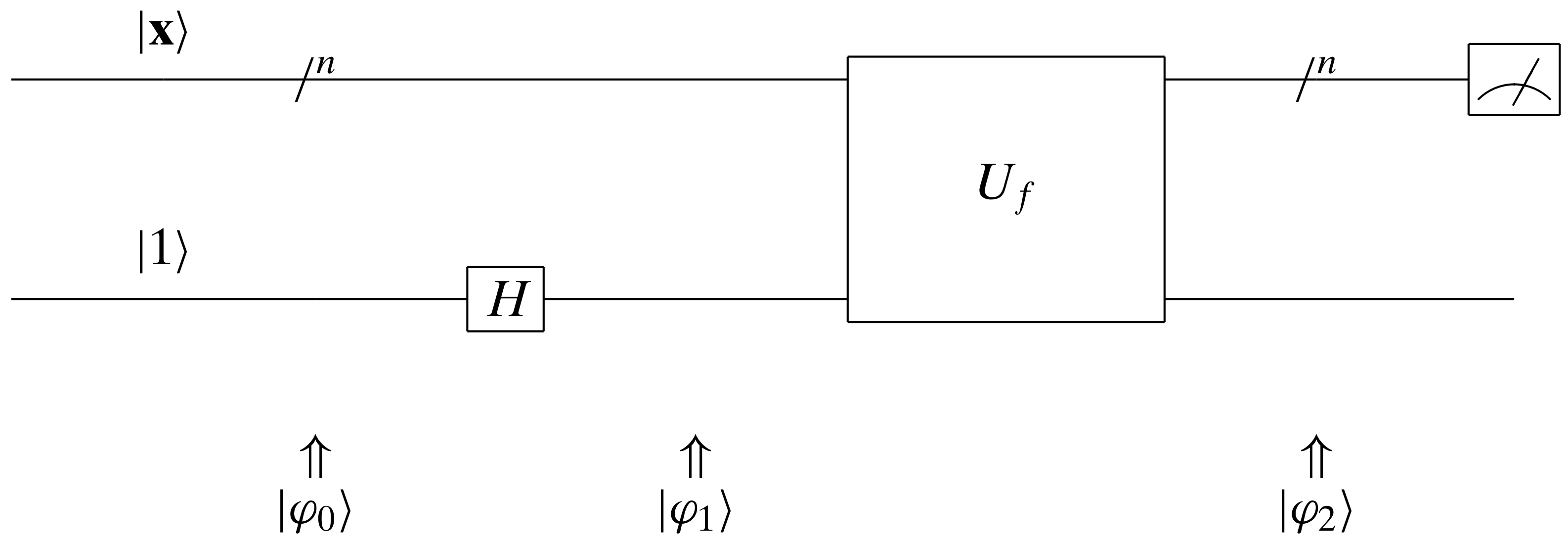This quantum oracle circuit has been well discussed in my previous article on Deutsch-Jozsa algorithm. We have known that

\begin{align} |\varphi_2\rangle &= (-1)^{f(\mathbf{x})} |\mathbf{x}\rangle \otimes \frac{|0\rangle - |1\rangle}{\sqrt{2}} \\ \end{align}

If the top input qubits to $U_f$ is an equal superposition, i.e.,

\begin{align} | \mathbf{s} \rangle &= H^{\otimes {n}} | \mathbf{0} \rangle \\ &= \frac{1}{\sqrt{2^n}} \sum_{ \mathbf{x} \in \{0,1\}^n } | \mathbf{x} \rangle \\ \end{align}

what will the top output qubits be?

Because we have assumed that there is only one $\mathbf{x} = \mathbf{x}^\ast$, such that $f(\mathbf{x}) = 1$, and for $\mathbf{x} \neq \mathbf{x}^\ast$ we have $f(\mathbf{x}) = 0$,

\begin{align} |\varphi_2\rangle &= \frac{1}{\sqrt{2^n}} \Bigg( \sum_{\mathbf{x} \neq \mathbf{x}^\ast}^{} (-1)^{0} |\mathbf{x}\rangle \otimes \frac{|0\rangle - |1\rangle}{\sqrt{2}} \Bigg) + \frac{1}{\sqrt{2^n}} (-1)^{1} |\mathbf{x}^\ast \rangle \otimes \frac{|0\rangle - |1\rangle}{\sqrt{2}} \\ &= \frac{1}{\sqrt{2^n}} \Bigg( \sum_{\mathbf{x} \neq \mathbf{x}^\ast}^{} |\mathbf{x}\rangle \otimes \frac{|0\rangle - |1\rangle}{\sqrt{2}} \Bigg) - \frac{1}{\sqrt{2^n}} |\mathbf{x}^\ast \rangle \otimes \frac{|0\rangle - |1\rangle}{\sqrt{2}} \\ &= \Bigg( \sum_{\mathbf{x} \neq \mathbf{x}^\ast}^{} \frac{1}{\sqrt{2^n}} |\mathbf{x}\rangle - \frac{1}{\sqrt{2^n}} |\mathbf{x}^\ast \rangle \Bigg) \otimes \frac{|0\rangle - |1\rangle}{\sqrt{2}} \\ \end{align}

This means that for the top output qubits, although we have equal probability to observe each of the base qubit states, the phase amplitude of the base qubit $|\mathbf{x}^\ast \rangle$ is different from all the others! That is also to say, the phase of the top input qubits is
$\big[ \frac{1}{\sqrt{2^n}}, \frac{1}{\sqrt{2^n}}, \cdots, \frac{1}{\sqrt{2^n}} \big]$, but the phase of the output qubits becomes $\big[ \frac{1}{\sqrt{2^n}}, \cdots, \frac{1}{\sqrt{2^n}}, -\frac{1}{\sqrt{2^n}}, \frac{1}{\sqrt{2^n}},\cdots, \frac{1}{\sqrt{2^n}} \big]$.

### Phase Diffusion Circuit

Now, in order to find $| \mathbf{x}^\ast \rangle$, the strategy becomes straightforward. We just have to find out the base qubits state whose phase amplitude is different from others. The question is, how to find it?

Let’s do some dummy trials. If we send the top output qubits to another identical quantum oracle circuit, the phase will go back to $\big[ \frac{1}{\sqrt{2^n}}, \frac{1}{\sqrt{2^n}}, \cdots, \frac{1}{\sqrt{2^n}} \big]$. The readers could verify this on you own. This means we have gone back to the origin. We should probably do something differently.

What Grover found was actually very smart. The average of the phase amplitudes $\big[ \frac{1}{\sqrt{2^n}}, \cdots, \frac{1}{\sqrt{2^n}}, -\frac{1}{\sqrt{2^n}}, \frac{1}{\sqrt{2^n}},\cdots, \frac{1}{\sqrt{2^n}} \big]$ is a value only a little bit smaller than $\frac{1}{\sqrt{2^n}}$, because the majority of the phase amplitudes are $\frac{1}{\sqrt{2^n}}$, and only one phase amplitude corresponding to $|\mathbf{x}^\ast \rangle$, which we denote as $\alpha_{\mathbf{x}^{\ast}}$, is $-\frac{1}{\sqrt{2^n}}$. If we could inverse the phase amplitude about the mean, the absolute value of $\alpha_{\mathbf{x}^{\ast}}$ will become the largest among all the phase amplitudes.

Specifically, at time step $t$, suppose the phase amplitude for each base qubits state $|\mathbf{x} \rangle$ is $\alpha_{\mathbf{x}, t}$. The average of the phase amplitudes $\mu_t$ is

$$\mu_t = \frac{1}{2^n} \sum_{\mathbf{x} \in \{0,1\}^n}^{} \alpha_{\mathbf{x}, t}$$

After the inversion about the mean, the phase amplitudes would become

\begin{align} \alpha_{\mathbf{x}, t}^{\prime} &= (\mu_t - \alpha_{\mathbf{x}, t}) + \mu_t \\ &= 2\mu_t - \alpha_{\mathbf{x}, t} \\ \end{align}

To do this in a matrix multiplication fashion, we denote $\boldsymbol{\mu}_t = [ \underbrace{\mu_t, \mu_t, \cdots, \mu_t}_{2^n} ]$.

\begin{align} \boldsymbol{\mu}_t &= \frac{1}{2^n} J_{2^n \times 2^n} \boldsymbol{\alpha}_{t} \\ &= A \boldsymbol{\alpha}_{t} \\ \end{align}

Where $A$ is the matrix we defined in the prerequisites.

\begin{align} \boldsymbol{\alpha}_{t}^{\prime} &= 2 \boldsymbol{\mu}_t - \boldsymbol{\alpha}_{t} \\ &= 2 A \boldsymbol{\alpha}_{t} - \boldsymbol{\alpha}_{t} \\ &= ( 2 A - I ) \boldsymbol{\alpha}_{t} \\ \end{align}

We notice that we have proved $2 A - I$ is a unitary matrix in the prerequisites, and therefore it could become a valid quantum gate!

Starting from time step $t = 0$, where the phase amplitudes are $\big[ \frac{1}{\sqrt{2^n}}, \cdots, \frac{1}{\sqrt{2^n}}, -\frac{1}{\sqrt{2^n}}, \frac{1}{\sqrt{2^n}},\cdots, \frac{1}{\sqrt{2^n}} \big]$. After the inversion about the mean, all the phase amplitudes have become positive and $\alpha_{\mathbf{x}^{\ast}}$ is the largest (slightly larger than all the others), which means that if we observe the top qubits we would have slightly larger chance of observing $|\mathbf{x}^\ast \rangle$. The problem is that the it is not large enough for the $|\mathbf{x}^\ast \rangle$ to be observed for sure. We would have to find out a way to further enhance $\alpha_{\mathbf{x}^{\ast}}$, the amplitude of $|\mathbf{x}^\ast \rangle$.

If we apply the top qubits to the quantum oracle again, the largest amplitude corresponding to $|\mathbf{x}^\ast \rangle$, $\alpha_{\mathbf{x}^{\ast}}$, would become negative. We further do inversion about the mean, $\alpha_{\mathbf{x}^{\ast}}$ would become positive again and it would become even larger. The phase amplitude amplification process could be illustrated using the following figures.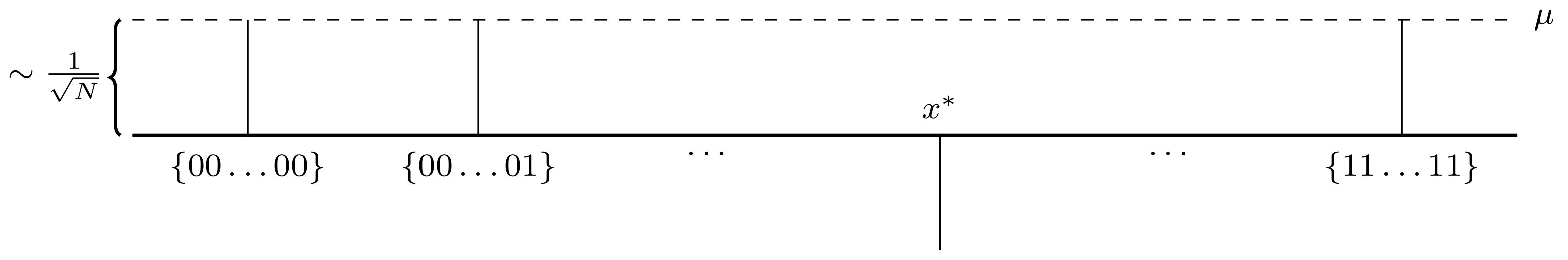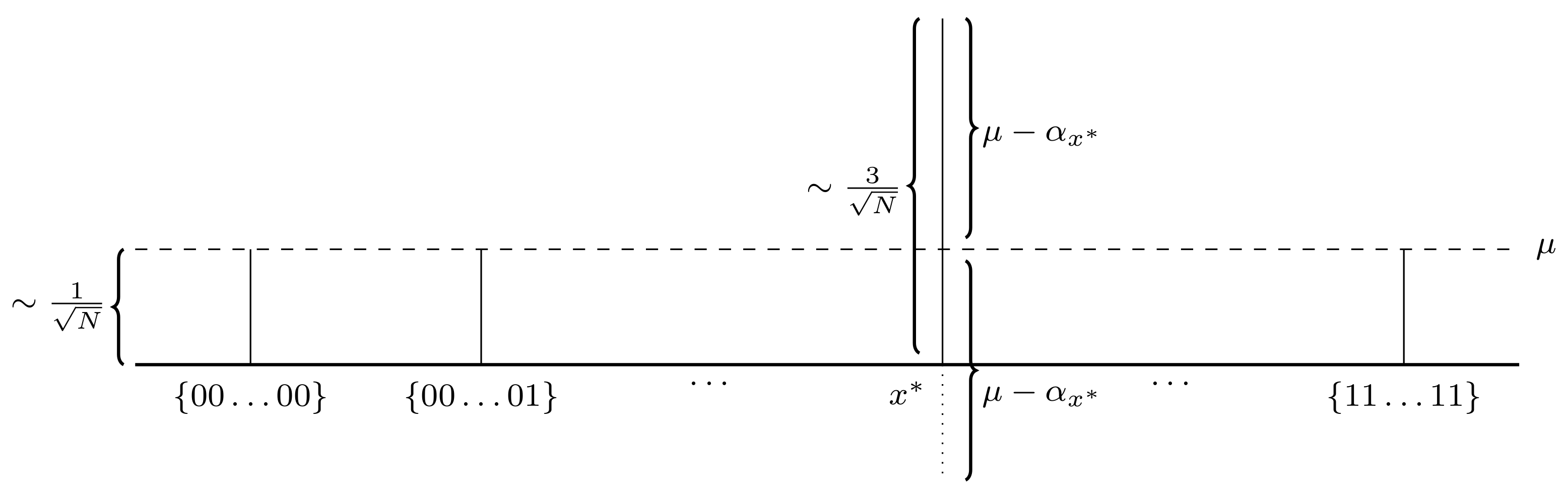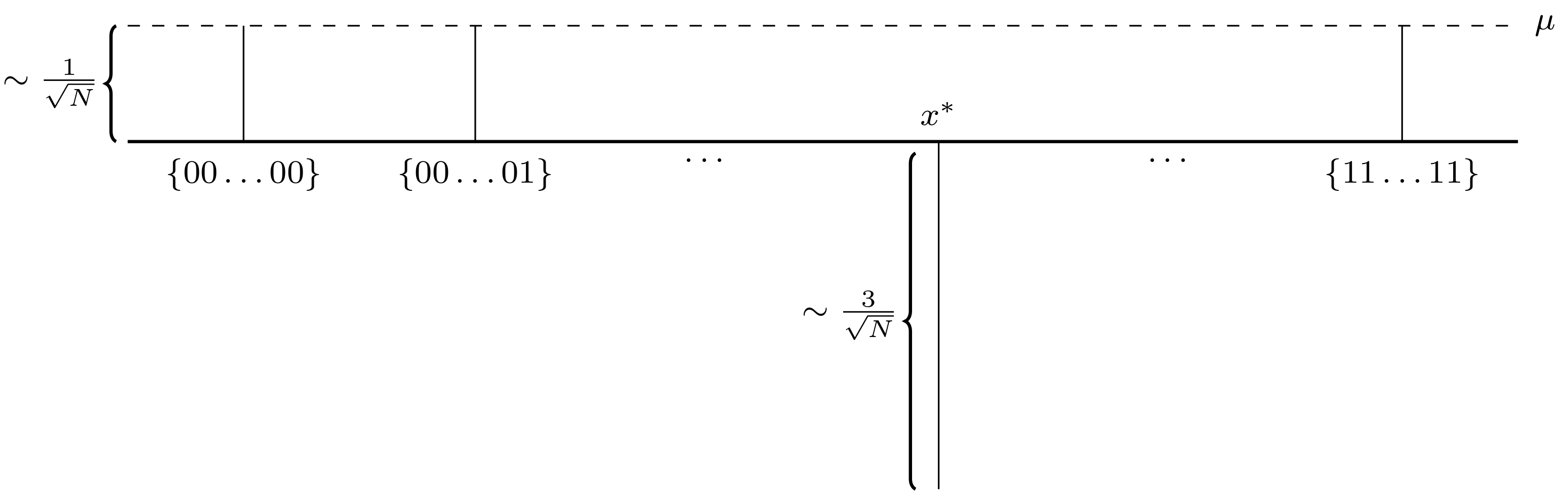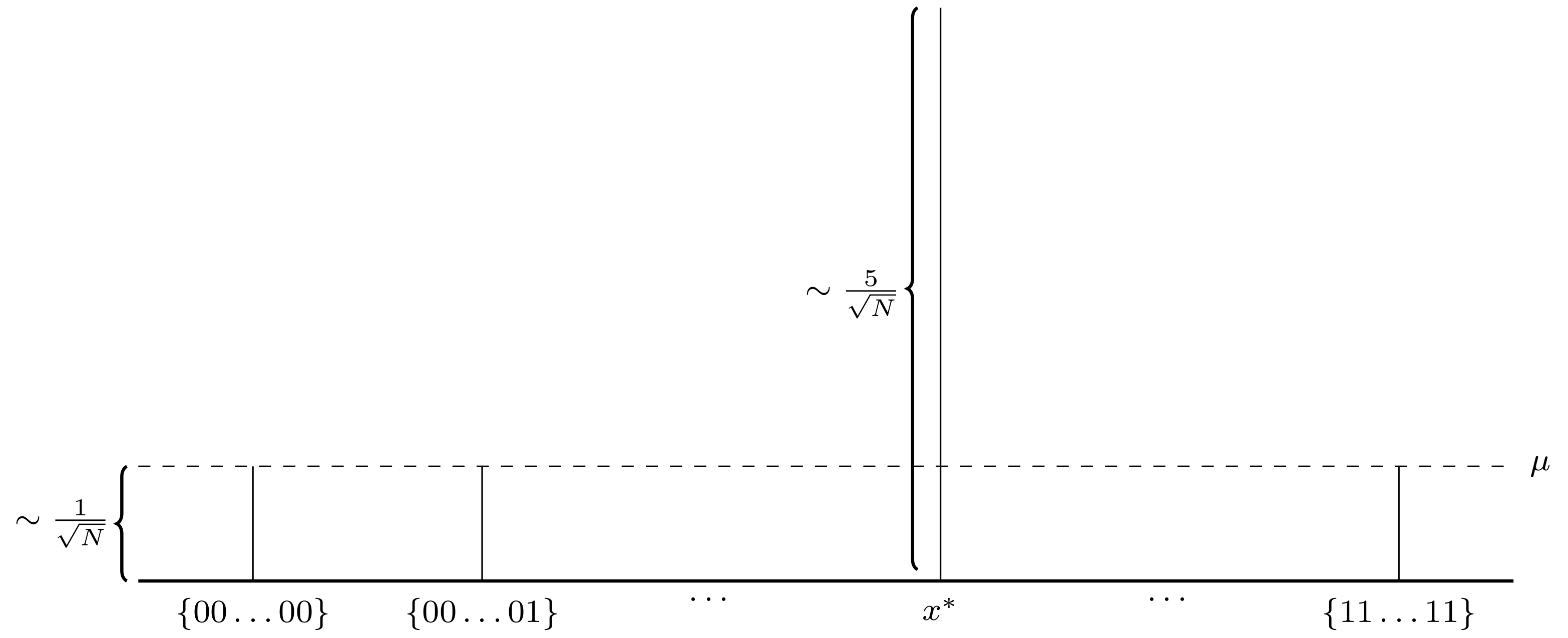In fact, with less than $O(\sqrt{2^n})$ iterations, we could make $\alpha_{\mathbf{x}^{\ast}}$ much larger than the rest of amplitudes so that we have nearly larger probability of observing $|\mathbf{x}^\ast \rangle$ from the top qubits. If we do more than $O(\sqrt{2^n})$ iterations, $\alpha_{\mathbf{x}^{\ast}}$ would become smaller and the probability of observing $|\mathbf{x}^\ast \rangle$ from the top qubits would become smaller. Let’s see how we could prove this.

A relatively trivial proof is first to prove

• $\alpha_{\mathbf{x}^{\ast}, t+1} \geq \alpha_{\mathbf{x}^{\ast}, t} + \frac{1}{\sqrt{2^n}}$ for $\alpha_{\mathbf{x}^{\ast}, t} \leq \frac{1}{2}$ and $n \neq 2$.
• $\alpha_{\mathbf{x}^{\ast}, t+1} \leq \alpha_{\mathbf{x}^{\ast}, t} + \frac{2}{\sqrt{2^n}}$ for any $t$.

followed by showing that

• $\alpha_{\mathbf{x}^{\ast}, \frac{\sqrt{2^n}}{8}} \geq 0.1$

These three proofs are trivial to show. The readers could try to derive them using the elemental algebra. If getting stuck, please refer to the lecture notes from CMU.

This means with exactly $\frac{\sqrt{2^n}}{8}$ iterations, the probability of observing $|\mathbf{x}^\ast \rangle$ from the top qubits is greater than $0.01$. Although $0.01$ is still not a high probability, we could repeat the process a constant number of times $k$, say $k = 1000$ times, we should expect that there is an observation that shows at least $10$ times among the $1000$ observations, which is significantly higher than the number of other individual observations. The most frequent observation would just be the $|\mathbf{x}^\ast \rangle$ that we are looking for. The number of query we have conducted is $\frac{k\sqrt{2^n}}{8}$, so asymptotically the query complexity is $O(\sqrt{2^n})$. $2^n$ is the total number of items $N$ in the database. We could also rewrite the query complexity to be $O(N)$.

There are two others ways to show that the query complexity is $O(\sqrt{2^n})$ which are much more elegant.

The most elegant proof is a geometric proof. It is the simplest proof regarding the form, but might be difficult for some of the readers who are not familiar with geometry and linear algebra.

Consider a plane spanned by $|\mathbf{s}\rangle$ and $|\mathbf{x}^{\ast}\rangle$, we have another vector

\begin{align} |\mathbf{s}^{\prime}\rangle &= \frac{1}{\sqrt{2^n - 1}} \sum_{\mathbf{x} \neq \mathbf{x}^\ast}^{} |\mathbf{x}\rangle \\ &= \frac{\sqrt{2^n}}{\sqrt{2^n - 1}} |\mathbf{s}\rangle - \frac{1}{\sqrt{2^n - 1}} |\mathbf{x}^{\ast}\rangle \\ \end{align}

Therefore, $|\mathbf{s}^{\prime}\rangle$ is also on the same plane.

In addition, $|\mathbf{s}^{\prime}\rangle$ and $|\mathbf{x}^{\ast}\rangle$ are orthogonal because

\begin{align} \langle \mathbf{s}^{\prime}, \mathbf{x}^{\ast}\rangle = 0 \end{align}

Applying the quantum oracle circuit only change the direction of the amplitude of $|\mathbf{x}^{\ast}\rangle$, and the amplitudes of the rest of $|\mathbf{x}\rangle$ remain unchanged. So it is a reflection about $|\mathbf{s}^{\prime}\rangle$.

In the first iteration, the reflection angle $\frac{\theta}{2}$ could be calculated using inner product.

\begin{align} \langle \mathbf{s}, \mathbf{s}^{\prime}\rangle &= \Bigg( \frac{1}{\sqrt{2^n}} \sum_{ \mathbf{x} \in \{0,1\}^n } | \mathbf{x} \rangle \Bigg) \Bigg( \frac{1}{\sqrt{2^n - 1}} \sum_{\mathbf{x} \neq \mathbf{x}^\ast}^{} |\mathbf{x}\rangle \Bigg) \\ &= \frac{1}{\sqrt{2^n}} \frac{1}{\sqrt{2^n - 1}} (2^n - 1) \\ &= \sqrt{\frac{2^n - 1}{2^n}} \\ &= |\mathbf{s}| |\mathbf{s}^{\prime}| \cos{\frac{\theta}{2}} \\ &= 1 \times 1 \cos{\frac{\theta}{2}} \\ &= \cos{\frac{\theta}{2}} \\ \end{align}

Therefore,

\begin{align} \cos{\frac{\theta}{2}} &= \sqrt{\frac{2^n - 1}{2^n}} \\ \sin{\frac{\theta}{2}} &= \frac{1}{\sqrt{2^n}} \\ \end{align}

When $n$ is large, $\frac{1}{\sqrt{2^n}}$ is a extremely small value, so we have

\begin{align} \sin{\frac{\theta}{2}} &= \frac{\theta}{2} \\ &= \frac{1}{\sqrt{2^n}} \\ \end{align}

So

$$\theta = \frac{2}{\sqrt{2^n}}$$

As we have discussed in the prerequisites, applying $2A - I$ to $|\mathbf{x}\rangle$ is reflecting $|\mathbf{x}\rangle$ about $|\mathbf{s}\rangle$. So here after reflecting $|\mathbf{s}\rangle$ about $|\mathbf{s}^{\prime}\rangle$, we further reflect the state vector about the original $|\mathbf{s}\rangle$. Wikipedia has a diagram illustrating this.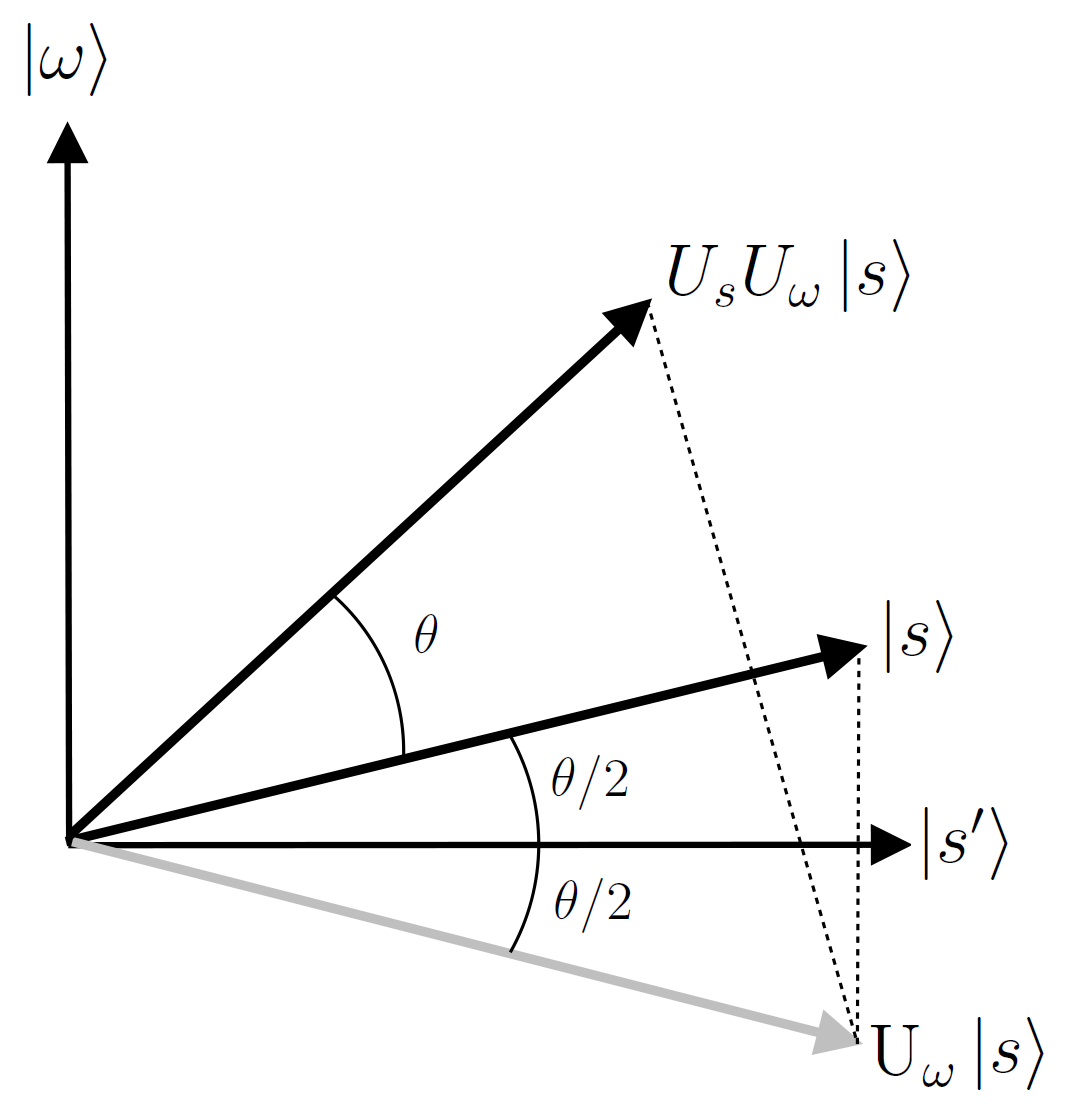Starting from a state vector which has an angle of $\frac{\pi - \theta}{2}$, after each iteration, the state vector moves angle $\theta$ closer to $|\mathbf{x}^{\ast}\rangle$, before it finally diverges from $|\mathbf{x}^{\ast}\rangle$.

The amplitude of $|\mathbf{x}^{\ast}\rangle$, $\alpha_{\mathbf{x}^{\ast}}$, is the projection of $|\mathbf{x}\rangle$ to $|\mathbf{x}^{\ast}\rangle$. In our case, the more close $|\mathbf{x}\rangle$ is to $|\mathbf{x}^{\ast}\rangle$ geometrically, the larger $\alpha_{\mathbf{x}^{\ast}}$ it is. Suppose the number of iterations we conducted is $k$, the angle the state vector and $|\mathbf{x}^{\ast}\rangle$ is $\frac{\pi}{2} - (k + \frac{1}{2})\theta$. The probability of observing $|\mathbf{x}^{\ast}\rangle$ from the top qubits is

\begin{align} \langle \mathbf{x}, \mathbf{w} \rangle^2 &= \cos^2 \bigg( \frac{\pi}{2} - (k + \frac{1}{2})\theta \bigg) \\ &= \sin^2\bigg( (k + \frac{1}{2})\theta \bigg) \\ \end{align}

To maximize the probability of observing $|\mathbf{x}^{\ast}\rangle$ from the top qubits, we have

\begin{align} (k + \frac{1}{2})\theta &= \frac{\pi}{2} \\ \end{align}

Therefore,

\begin{align} k &= \frac{\pi}{2\theta} - \frac{1}{2} \\ &\approx \frac{\pi \sqrt{2^n}}{4} - \frac{1}{2} \\ &\approx \frac{\pi \sqrt{2^n}}{4}\\ \end{align}

Therefore, the asymptotically the query complexity is $O(\sqrt{2^n})$.

This concludes the proof.

There is another algebraic proof which could be found on Wikipedia. The readers could refer to it if interested in. I will skip the formal proof in this article.

### Grover’s Algorithm

To summarize, Grover’s algorithm could be represented using the following diagram. To find an exact match to the query from the database, we only have to run Grover’s algorithm $O(2^n)$ times.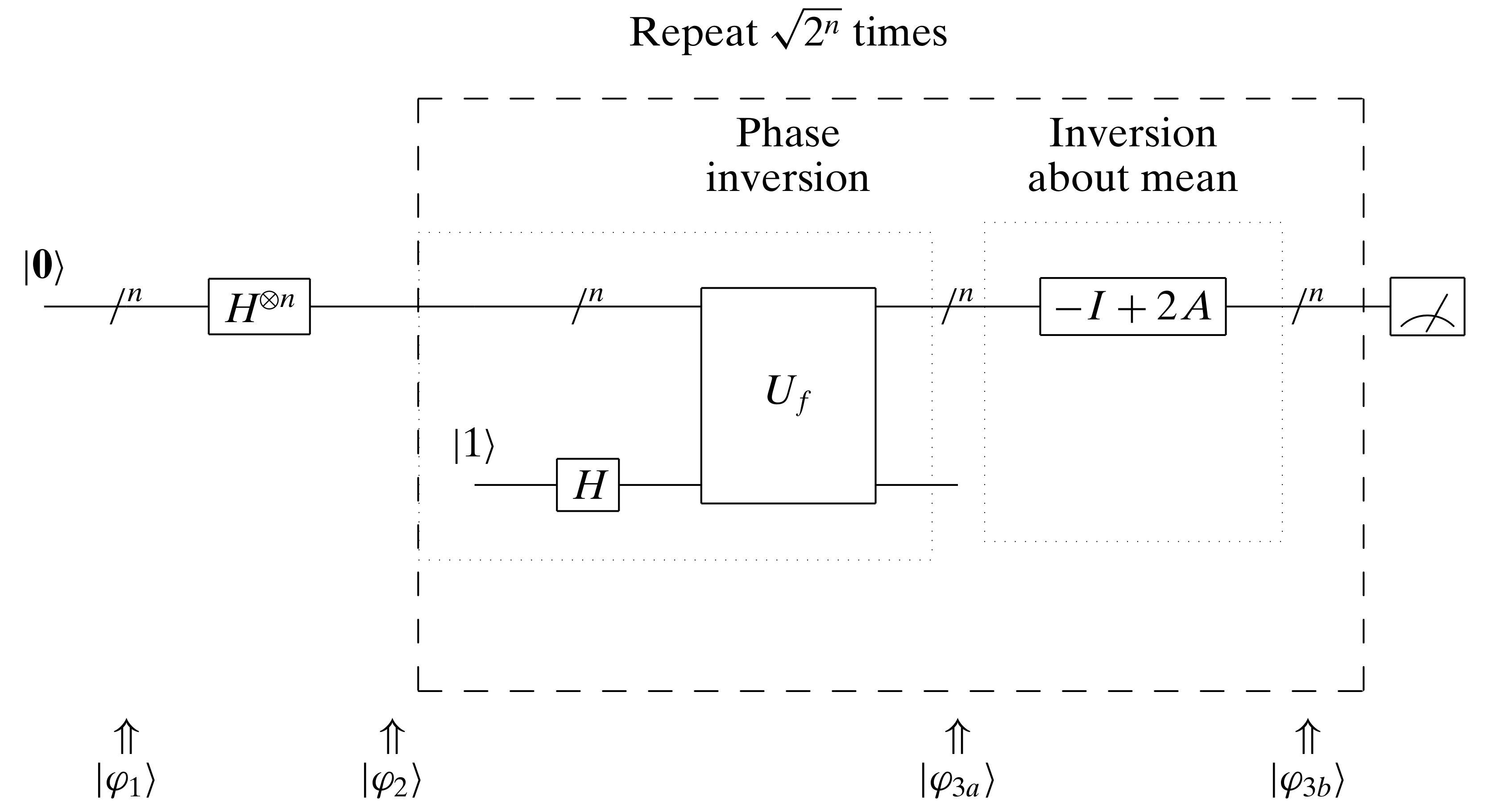### Quantum Circuit High-Level Design

If we treat the quantum oracle circuit as a quantum gate $U_x$ (not $U_f$ but including $U_f$) that takes in $n$ qubits and outputs $n$ qubits, to design the quantum oracle circuit for the identity query,

\begin{align} f(\mathbf{x}) &= \begin{cases} 1 & \text{when \mathbf{x} = \mathbf{x}^\ast}\\ 0 & \text{when \mathbf{x} \neq \mathbf{x}^\ast}\\ \end{cases} \end{align}

$U_x$ must have the information of $\mathbf{x}^\ast$.

$$U_x = I - 2 | \mathbf{x}^\ast \rangle \langle \mathbf{x}^\ast |$$

It is easy to verify that $U_x$ is unitary and flipping the amplitude of $| \mathbf{x}^\ast \rangle$.

\begin{align} U_x | \mathbf{x}^\ast \rangle &= - | \mathbf{x}^\ast \rangle \\ U_x | \mathbf{x} \rangle &= | \mathbf{x} \rangle \qquad \forall \mathbf{x} \neq \mathbf{x}^\ast \\ \end{align}

The design of the quantum diffusion circuit has been discussed earlier in the prerequisites, which is $2 | \mathbf{s} \rangle \langle \mathbf{s} | - I$.

### More Than One Matches

In the above derivations, we have an assumption that there is only one item matching the query in the database. What if we have multiple items matching the query in the database? Suppose there are $k$ items matching the query in the database, what is the asymptotical query complexity of finding one item matching the query from the database?

We extended the Grover’s algorithm geometric proof. Suppose, the $k$ matching items are $S$ = $\{ |\mathbf{x}_{1}^{\ast}\rangle$, $|\mathbf{x}_{2}^{\ast}\rangle$, $\cdots$, $|\mathbf{x}_{k}^{\ast}\rangle \}$.

We define two vectors.

\begin{align} |\mathbf{s}^{\prime}\rangle &= \frac{1}{\sqrt{2^n - k}} \sum_{\mathbf{x} \notin S} |\mathbf{x}\rangle \\ |\mathbf{s}^{\prime\prime}\rangle &= \frac{1}{\sqrt{k}} \sum_{\mathbf{x} \in S} |\mathbf{x}\rangle \\ \end{align}

For any $i \in [1,k]$, Consider a plane spanned by $|\mathbf{s}\rangle$ and $|\mathbf{s}^{\prime\prime}\rangle$, $|\mathbf{s}^{\prime}\rangle$ is also on the same plane, because

$$|\mathbf{s}^{\prime}\rangle = |\mathbf{s}\rangle - |\mathbf{s}^{\prime\prime}\rangle$$

Therefore, $|\mathbf{s}^{\prime}\rangle$ is also on the same plane.

In addition, $|\mathbf{s}^{\prime}\rangle$ and $|\mathbf{s}^{\prime\prime}\rangle$ are orthogonal because

\begin{align} \langle \mathbf{s}^{\prime}, \mathbf{s}^{\prime\prime}\rangle = 0 \end{align}

Similar to what we did previously,

\begin{align} \langle \mathbf{s}, \mathbf{s}^{\prime}\rangle &= \Bigg( \frac{1}{\sqrt{2^n}} \sum_{ \mathbf{x} \in \{0,1\}^n } | \mathbf{x} \rangle \Bigg) \Bigg( \frac{1}{\sqrt{2^n - k}} \sum_{\mathbf{x} \notin S}^{} |\mathbf{x}\rangle \Bigg) \\ &= \frac{1}{\sqrt{2^n}} \frac{1}{\sqrt{2^n - k}} (2^n - k) \\ &= \sqrt{\frac{2^n - k}{2^n}} \\ &= |\mathbf{s}| |\mathbf{s}^{\prime}| \cos{\frac{\theta}{2}} \\ &= 1 \times 1 \cos{\frac{\theta}{2}} \\ &= \cos{\frac{\theta}{2}} \\ \end{align}

Therefore,

\begin{align} \cos{\frac{\theta}{2}} &= \sqrt{\frac{2^n - k}{2^n}} \\ \sin{\frac{\theta}{2}} &= \sqrt{\frac{k}{2^n}} \\ \end{align}

\begin{align} \sin{\frac{\theta}{2}} &\approx \frac{\theta}{2} \\ &\approx \sqrt{\frac{k}{2^n}} \\ \end{align}

$$\theta \approx 2\sqrt{\frac{k}{2^n}}$$

\begin{align} \langle \mathbf{x}, \mathbf{w} \rangle^2 &= \cos^2 \bigg( \frac{\pi}{2} - (k + \frac{1}{2})\theta \bigg) \\ &= \sin^2\bigg( (k + \frac{1}{2})\theta \bigg) \\ \end{align}

To maximize the probability of observing one of the $S$ = $\{ |\mathbf{x}_{1}^{\ast}\rangle$, $|\mathbf{x}_{2}^{\ast}\rangle$, $\cdots$, $|\mathbf{x}_{k}^{\ast}\rangle \}$, from the top qubits, we have

\begin{align} (k + \frac{1}{2})\theta &= \frac{\pi}{2} \\ \end{align}

Therefore,

\begin{align} k &= \frac{\pi}{2\theta} - \frac{1}{2} \\ &\approx \frac{\pi \sqrt{\frac{2^n}{k}}}{4} - \frac{1}{2} \\ &\approx \frac{\pi \sqrt{\frac{2^n}{k}}}{4}\\ \end{align}

Therefore, the asymptotically the query complexity is $O(\sqrt{\frac{2^n}{k}})$. As long as $1 \leq k \ll 2^n$, the asymptotically the query complexity is still $O(\sqrt{2^n})$

This concludes the proof. The entire proof is very similar to the one for the single one targe assumption one. In fact, the single one targe assumption one is just a special case of this more general one.

## Practical Grover’s Algorithm

It seems that there is something unusual for Grover’s algorithm. Since the quantum oracle circuit already has the target index information, and the designer must have known that, why the fuck do we have to run the Grover’s algorithm?! Is Grover’s algorithm actually useful in practice?! I think people got confused because Grover’s algorithm was designed for querying an unstructured database instead of a structured database, and the databases we commonly know are structured database. As we have seen, the input to Grover’s algorithm is a superposition of $2^n$ base states, and this is an unstructured database containing $2^n$ items. Common structured databases uses tree structure or hashing, but unstructured database stores data linearly. While the asymptotical query complexity of the algorithms that we commonly use for structured database are $O(\log{N})$ for tree-structured database and $O(1)$ for hashing based database, the asymptotical query complexity of the classical algorithms for unstructured database is $O(N)$.

### Possible Useful Scenario

OK, this did not answer why we have to run the Grover’s algorithm. In fact, querying something that is identical to the ones in the unstructured database by running Grover’s algorithm does not make sense, since we have to know where the identical ones are in the unstructured database in order to design the quantum oracle circuits. What’s making Grover’s algorithm powerful is that by carefully designing the quantum oracle circuits, we could actually query something that matches the patterns we specified!

For example, instead of having a simple query $\mathbf{x} = \mathbf{x}^\ast$,

\begin{align} f(\mathbf{x}) &= \begin{cases} 1 & \text{when \mathbf{x} = \mathbf{x}^\ast}\\ 0 & \text{when \mathbf{x} \neq \mathbf{x}^\ast}\\ \end{cases} \end{align}

We could design a more sophisticated query for some patterns that the quantum oracle circuit does not know the exact answers, for example,

\begin{align} f(\mathbf{x}) &= \begin{cases} 1 & \text{when \mathbf{x}_{0:\frac{n}{2}} + \mathbf{x}_{\frac{n}{2}:n} = \mathbf{w}^\ast}\\ 0 & \text{when \mathbf{x}_{0:\frac{n}{2}} + \mathbf{x}_{\frac{n}{2}:n} \neq \mathbf{w}^\ast}\\ \end{cases} \end{align}

where $\mathbf{x}_{0:\frac{n}{2}}$ is the first half of the bits, $\mathbf{x}_{\frac{n}{2}:n}$ is the second half of the bits, and $\mathbf{w}^\ast$ is the query bits.

Using a classical search algorithm, it would take $O(N)$ to go through the entire unstructured database. Using Grover’s algorithm, as long as the number of targets in the database is significantly smaller than $\sqrt{N}$, we could always find all of them by running Grover’s algorithm $O(\sqrt{N})$ times. However, designing such quantum oracle circuit might not be as trivial as the one that used for query the identical match which we have discussed early in this article.

More generally, if we could design quantum oracle circuits that does

\begin{align} f(\mathbf{x}) &= \begin{cases} 1 & \text{when g(\mathbf{x}) = \mathbf{w}^\ast}\\ 0 & \text{when g(\mathbf{x}) \neq \mathbf{w}^\ast}\\ \end{cases} \end{align}

for any specific $g$, and the number of targets in the database is significantly smaller than $\sqrt{N}$, we could always find all of them by running Grover’s algorithm $O(\sqrt{N})$ times.

## Final Remarks

In practice, Grover’s algorithm is not as useful as what I expected. But I could be short-sighted.

## Miscellaneous

Where is Lov Grover right now? Was not able to find him Bell Labs anymore. Have not heard any news from him in recent years.